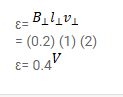# A metallic meter stick moves with a velocity of

Question:

A metallic meter stick moves with a velocity of $2 \mathrm{~m} / \mathrm{s}$ in a direction perpendicular to its length and perpendicular to a uniform magnetic field of magnitude $0.2 \mathrm{~T}$. Find the emf induced between the ends of the stick.

Solution: